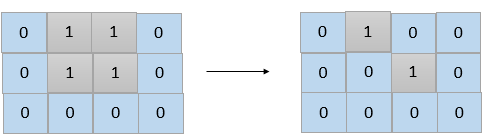Formatted question description: https://leetcode.ca/all/1568.html

# 1568. Minimum Number of Days to Disconnect Island (Medium)

Given a 2D grid consisting of 1s (land) and 0s (water).  An island is a maximal 4-directionally (horizontal or vertical) connected group of 1s.

The grid is said to be connected if we have exactly one island, otherwise is said disconnected.

In one day, we are allowed to change any single land cell (1) into a water cell (0).

Return the minimum number of days to disconnect the grid.

Example 1:Input: grid = [[0,1,1,0],[0,1,1,0],[0,0,0,0]]
Output: 2
Explanation: We need at least 2 days to get a disconnected grid.
Change land grid and grid to water and get 2 disconnected island.


Example 2:

Input: grid = [[1,1]]
Output: 2
Explanation: Grid of full water is also disconnected ([[1,1]] -> [[0,0]]), 0 islands.


Example 3:

Input: grid = [[1,0,1,0]]
Output: 0


Example 4:

Input: grid = [[1,1,0,1,1],
[1,1,1,1,1],
[1,1,0,1,1],
[1,1,0,1,1]]
Output: 1


Example 5:

Input: grid = [[1,1,0,1,1],
[1,1,1,1,1],
[1,1,0,1,1],
[1,1,1,1,1]]
Output: 2


Constraints:

• 1 <= grid.length, grid[i].length <= 30
• grid[i][j] is 0 or 1.

Related Topics: Greedy

## Solution 1.

You need at most two days to separate out a 1 at the corner with all other 1s. So the answer is one of 0, 1, 2.

If the graph is already disconnected, return 0.

For each 1, see if removing it can disconnect the graph. If yes, then return 1.

Otherwise return 2.

// OJ: https://leetcode.com/problems/minimum-number-of-days-to-disconnect-island/

// Time: O((MN)^2)
// Space: O(MN)
class Solution {
int M, N, dirs = { {0,1},{0,-1},{1,0},{-1,0} };
void dfs(vector<vector<int>> &G, int i, int j,vector<vector<int>> &seen) {
seen[i][j] = true;
for (auto &[dx, dy] : dirs) {
int x = dx + i, y = dy + j;
if (x < 0 || x >= M || y < 0 || y >= N || G[x][y] != 1 || seen[x][y]) continue;
dfs(G, x, y, seen);
}
}
bool disconnected(vector<vector<int>> &G) {
vector<vector<int>> seen(M, vector<int>(N, false));
int cnt = 0;
for (int i = 0; i < M; ++i) {
for (int j = 0; j < N; ++j) {
if (G[i][j] != 1 || seen[i][j]) continue;
if (++cnt > 1) return true;
dfs(G, i, j, seen);
}
}
return cnt == 0;
}
public:
int minDays(vector<vector<int>>& G) {
M = G.size(), N = G.size();
if (disconnected(G)) return 0;
for (int i = 0; i < M; ++i) {
for (int j = 0; j < N; ++j) {
if (G[i][j] != 1) continue;
G[i][j] = 0;
if (disconnected(G)) return 1;
G[i][j] = 1;
}
}
return 2;
}
};


Java

class Solution {
int[][] directions = { {-1, 0}, {1, 0}, {0, -1}, {0, 1} };

public int minDays(int[][] grid) {
int lands = 0;
int count = 0;
int minConnection = 4;
int rows = grid.length, columns = grid.length;
int[][] connections = new int[rows][columns];
boolean[][] visited = new boolean[rows][columns];
for (int i = 0; i < rows; i++) {
for (int j = 0; j < columns; j++) {
if (grid[i][j] == 1) {
lands++;
int curConnection = getConnection(grid, i, j);
connections[i][j] = curConnection;
minConnection = Math.min(minConnection, curConnection);
if (!visited[i][j]) {
count++;
depthFirstSearch(grid, visited, i, j);
}
}
}
}
if (count > 1)
return 0;
else if (minConnection == 1)
return lands == 2 ? 2 : 1;
else {
for (int i = 0; i < rows; i++) {
for (int j = 0; j < columns; j++) {
if (grid[i][j] == 1 && canDisconnect(grid, i, j))
return 1;
}
}
return 2;
}
}

public int getConnection(int[][] grid, int row, int column) {
int connection = 0;
int rows = grid.length, columns = grid.length;
for (int[] direction : directions) {
int newRow = row + direction, newColumn = column + direction;
if (newRow >= 0 && newRow < rows && newColumn >= 0 && newColumn < columns && grid[newRow][newColumn] == 1)
connection++;
}
return connection;
}

public boolean canDisconnect(int[][] grid, int row, int column) {
grid[row][column] = 0;
int count = 0;
int rows = grid.length, columns = grid.length;
boolean[][] visited = new boolean[rows][columns];
for (int i = 0; i < rows; i++) {
for (int j = 0; j < columns; j++) {
if (grid[i][j] == 1 && !visited[i][j]) {
if (count == 0) {
count++;
depthFirstSearch(grid, visited, i, j);
} else {
grid[row][column] = 1;
return true;
}
}
}
}
grid[row][column] = 1;
return false;
}

public void depthFirstSearch(int[][] grid, boolean[][] visited, int row, int column) {
visited[row][column] = true;
int rows = grid.length, columns = grid.length;
for (int[] direction : directions) {
int newRow = row + direction, newColumn = column + direction;
if (newRow >= 0 && newRow < rows && newColumn >= 0 && newColumn < columns && grid[newRow][newColumn] == 1 && !visited[newRow][newColumn])
depthFirstSearch(grid, visited, newRow, newColumn);
}
}
}